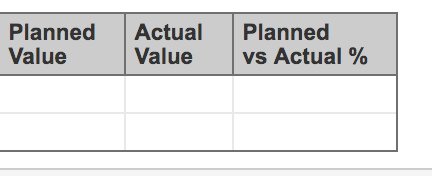# Help how to create a measure that calculates a total and a %

I am a complete newbie to Eazy Bi! So apologies if this is a little basic.

I have 2 measures that I defined 'Planned Value and ‘Actual Value’ which I calculated by the following:
Planned Value:
`[Issue].CurrentMember.get('Planned Value')`
&
Actual Value:
`[Issue].CurrentMember.get('Actual Value')`

What I need is to create first a measure that takes each value and adds it up to a total so rather than getting a set of say 1,2,5 I want it to return 8. So I am having trouble making this calculation, any pointers.Then once I have a total for each measure I need to calculate what % of the planned value was achieved by the actual value.

It might be worth mentioning the fields return a string.

Any guidance or starting points appreciated!

Hi,

your formulas of the Planned and Actual value refers to the Issue properties. I assume those formulas should work fine, if you have imported the respective numeric custom fields as properties. Note, that the properties of issues works only at the Issue level, those are not the measures that aggregate the values when you need the totals.

For the totals, you need to import the custom fields also as measures:

Such options would create a set of measures with different mapping to the time dimension.
You should use the “Planned value created” and “Actual value created” measures from the custom field section for calculation of the % of earned value calculation in your report:

Please, check the documentation for more about measures and properties in eazyBI:
https://docs.eazybi.com/eazybijira/getting-started/measures-and-dimensions

Kindly,

1 Like

Hi thank you for you reply Janis!
In Jira the Planned Value and Actual Value are actually dimensions when I imported the data there wasn’t an option to import as a measure only as a dimension. So I created a custom measures listed above e.g.
`[Issue].CurrentMember.get('Planned Value')`

This seems to work ok on each line item as well as the % calculation but none of the calculations roll up to the level above.

Thank you for the link, I will take a look and see if I can import the custom fields.